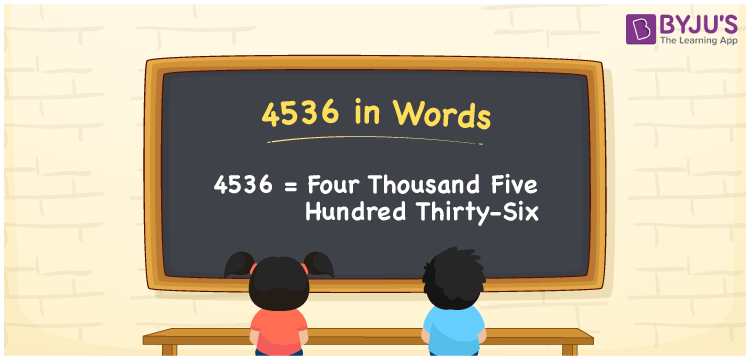We can write 4536 in words as Four Thousand Five Hundred Thirty-six. Suppose you have Rs. 4536 in your bank account, you can say that “I have Four Thousand Five Hundred Thirty-six rupees in my bank account”. It is possible to convert 4536 into words with the help of a place value chart. Also, we know that 4536 is a cardinal number since it represents a specific quantity.

 4536 in words Four Thousand Five Hundred Thirty-six Four Thousand Five Hundred Thirty-six in Numbers 4536

## 4536 in English Words## How to Write 4536 in Words?

We can convert 4536 to words using a place value chart. This can be done as follows. The number 4536 has 4 digits, so let’s make a chart that shows the place value up to 4 digits.

 Thousands Hundreds Tens Ones 4 5 3 6

Thus, we can write the expanded form as:

4 × Thousand + 5 × Hundred + 3 × Ten + 6 × One

= 4 × 1000 + 5 × 100 + 3 × 10 + 6 × 1

= 4536

= Four Thousand Five Hundred Thirty-six

4536 is the natural number that is succeeded by 4535 and preceded by 4537.

4536 in words – Four Thousand Five Hundred Thirty-six

Is 4536 an odd number? – No.

Is 4536 an even number? – Yes

Is 4536 a perfect square number? – No

Is 4536 a perfect cube number? – No

Is 4536 a prime number? – No

Is 4536 a composite number? – Yes

## Frequently Asked Questions on 4536 in Words

Q1

### How to write 4536 in words?

4536 in English is written as “Four Thousand Five Hundred Thirty-six”.
Q2

### Is 4536 a prime number?

No, the number 4536 is not a prime number. Four Thousand Five Hundred Thirty-six is a composite number. Because 4536 has more divisors than 1 and itself.
Q3

### Write Four Thousand Five Hundred Thirty-six in numbers.

Four Thousand Five Hundred Thirty-six in numbers is 4536.